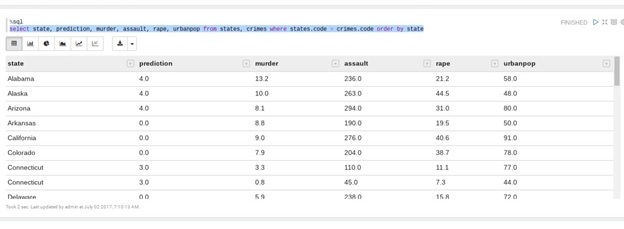﻿ K-means Clustering with Apache Spark – BMC Software | Blogs
Machine Learning & Big Data Blog

# K-means Clustering with Apache SparkHere we show a simple example of how to use k-means clustering. We will look at crime statistics from different states in the USA to show which are the most and least dangerous.

We get our data from here.

(This tutorial is part of our Apache Spark Guide. Use the right-hand menu to navigate.)

The data looks like this. The columns are state, cluster, murder rate, assault, population, and rape. It already includes a cluster column which we will drop. That is because you can cluster data points into as many clusters as you like. This data set used some other value. Also notice that the population is a normalized value, meaning it is not the actual population. This makes it a small scale number, which is a common approach to statistics.

Delete the column headings in order to read in the data. Later we will put them back.

crime\$cluster Murder Assault UrbanPop Rape
Alabama 4 13.2 236 58 21.2
Alaska 4 10 263 48 44.5
Arizona 4 8.1 294 80 31
Arkansas 3 8.8 190 50 19.5
California 4 9 276 91 40.6
Colorado 3 7.9 204 78 38.7

Below is the Scala code. We paste it into a Zeppelin notebook since that does graphs nicely. (You can read about using Zeppelin with Apache Spark in a previous post we wrote here.)

First we have our normal imports:

```import org.apache.spark.mllib.clustering.{KMeans, KMeansModel}
import org.apache.spark.mllib.linalg.Vectors
import sqlContext.implicits._
import org.apache.spark.sql.types._```

Then we create the crime data rdd from the crime_data.csv file, which we copied to Hadoop.

`var rdd = sc.textFile("hdfs://localhost:9000/data/crime_data.csv")`

Now, data sent to a machine learning algorithm has to be numbers. So this algorithm will convert a string to bytes and then to a double. Then we loop across each byte group and sum them to make a single total number that will be the encoded value of the text field state.

```def stoDouble (s : String): Double = {
return s.map(_.toByte.doubleValue()).reduceLeft( (x,y) => x + y)
}```

We obviously need to translate that number back into text so we can see what state we are dealing with. So we make a case class. You do that when you want to create a dataframe. You create a dataframe when you want to use SQL, which is easy to work with.

```case class StateCode(State:String, Code:Double)
var lines = rdd.map(l => l.split(","))
var states = lines.map(l => StateCode(l(0),stoDouble(l(0)))).toDF()
states.show()
states.createOrReplaceTempView("states")```

Here we take each line in the input data file and make it an array of doubles.

```def makeDouble (s: String): Array[Double] = {
var str = s.split(",")
var a = stoDouble(str(0))
return Array(a,str(2).toDouble,str(3).toDouble,str(4).
toDouble,str(5).toDouble)
}
var crime = rdd.map(m => makeDouble(m))```

Now we make an object called a Dense Vector. The Spark k-means classification algorithm requires that format. Then we run the train method to cause the machine learning algorithm to group the states into clusters based upon the crime rates and population. We tell it to use five clusters. We could have said 10 or any number. The larger the number of clusters, the more you have divided your data.

```val crimeVector = crime.map(a => Vectors.dense(a(0),a(1),a(2),a(3),a(4)))
val clusters = KMeans.train(crimeVector,5,10)```

Now we create another case class so that the end results will be in a data frame with names columns. Here we have all the date plus the Dense Vector object as well the prediction that Spark will make based upon the k-means algorithm. A point to note here is that Spark has no problem in making a dense vector an SQL column. Notice that the clusters value from the training set is used to make the prediction. We add that to the Crime case class as well.

```case class Crime (Code:Double, Murder:Double, Assault:Double, UrbanPop:Double,Rape:Double, PredictionVector:org.apache.spark.mllib.linalg.Vector, Prediction:Double)
val crimeClass = crimeVector.map(a => Crime(a(0), a(1), a(2), a(3), a(4), a ,clusters.predict(a))).toDF()
crimeClass.show()
crimeClass.createOrReplaceTempView("crimes")```

Finally, we have to join the two tables of state and prediction because we want to be able to show the state name and not the number we converted it too. Notice the %sql line. In a Zeppelin notebook that means we want to use SQL. We can do that because in crimeClass.createOrReplaceTempView(“crimes”) we created a temporary view that we can query with SQL. When you use %sql Zeppelin will automatically give you the option to create different graphs.

```%sql
select state, prediction, murder, assault, rape, urbanpop from states, crimes where states.code = crimes.code order by state```

Here we output the option as a table. We could have made a pie chart, bar graph, or other. As you can see, stay away from Alabama as it is more dangerous than, for example, Colorado.This e-book teaches machine learning in the simplest way possible. This book is for managers, programmers, directors – and anyone else who wants to learn machine learning. We start with very basic stats and algebra and build upon that.

These postings are my own and do not necessarily represent BMC's position, strategies, or opinion.

See an error or have a suggestion? Please let us know by emailing blogs@bmc.com.

### BMC Bring the A-Game

From core to cloud to edge, BMC delivers the software and services that enable nearly 10,000 global customers, including 84% of the Forbes Global 100, to thrive in their ongoing evolution to an Autonomous Digital Enterprise.